Importance: Medium ✭✭
 Author(s): Noel, Jonathan A.
 Subject: Graph Theory » Coloring » » Vertex coloring
 Keywords: choosability complete multipartite graph list coloring
 Posted by: Jon Noel on: February 2nd, 2013
Conjecture   Ifis a-chromatic graph on at mostvertices, then.

For integers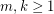, let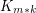denote the complete-partite graph in which every part has size.

In one of the original papers on choosability, Erdos, Rubin and Taylor [ERT] proved that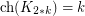. Later, Ohba [Ohba] conjectured the following generalization: if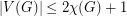, then TeX Embedding failed!.} This was proved by Noel, Reed and Wu [NRW12].

Theorem  (Noel, Reed and Wu 2012)   If, then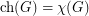.

The above theorem implies that the above conjecture holds for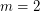. That is, ifis a-chromatic graph on at most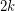vertices (in fact, at most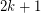vertices), then.

Kierstead [Kie00] proved that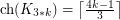. This was generalized by Noel, West, Wu and Zhu [NWWZ13] to the following:

Theorem  (Noel, West, Wu and Zhu 2013)   For every graph,Therefore, ifis a-chromatic graph on at mostvertices, then. This shows that the conjecture is true for.

Recently, Kierstead, Salmon and Wang [KSW14] proved the following:

Theorem  (Kierstead, Salmon and Wang 2014)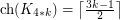.

However, it is not known whether the upper bound of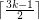holds for all-chromatic graphs on at most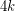vertices. If true, it would verify the conjecture for.

The following is a refinement of the conjecture.

Conjecture  (Noel 2013)   Forthere is a graphsuch that
\itemis a complete-partite graph onvertices, \item the stability number ofis, and \item every-chromatic graphon at mostvertices satisfies.

## Bibliography

[Alo92] N. Alon. Choice numbers of graphs: a probabilistic approach. Combin. Probab. Comput., 1(2):107–114, 1992.

[ERT80] P. Erdos, A. L. Rubin, and H. Taylor. Choosability in graphs. Congress. Numer., XXVI, pages 125–157, 1980.

[Kie00] H. A. Kierstead. On the choosability of complete multipartite graphs with part size three. Discrete Math., 211(1-3):255–259, 2000.

[KSW14] H. A. Kierstead, A. Salmon and R. Wang. On the Choice Number of Complete Multipartite Graphs With Part Size Four.

*[Noe13] J. A. Noel. Choosability of Graphs With Bounded Order: Ohba's Conjecture and Beyond. Master's thesis, McGill University, Montreal. pdf

[NRW12] J. A. Noel, B. A. Reed, and H. Wu. A Proof of a Conjecture of Ohba. Preprint, arXiv:1211.1999v1, November 2012. Webpage

[NWWZ13] J. A. Noel, D. B. West, H. Wu, and X. Zhu. Beyond Ohba's Conjecture: A bound on the choice number of-chromatic graphs withvertices. Preprint, arXiv:1308.6739v1, August 2013. pdf

[Ohb02] K. Ohba. On chromatic-choosable graphs. J. Graph Theory, 40(2):130–135, 2002.

[Yan03] D. Yang. Extension of the game coloring number and some results on the choosability of complete multipartite graphs. PhD thesis, Arizona State University, Tempe, Arizona, 2003.

* indicates original appearance(s) of problem.